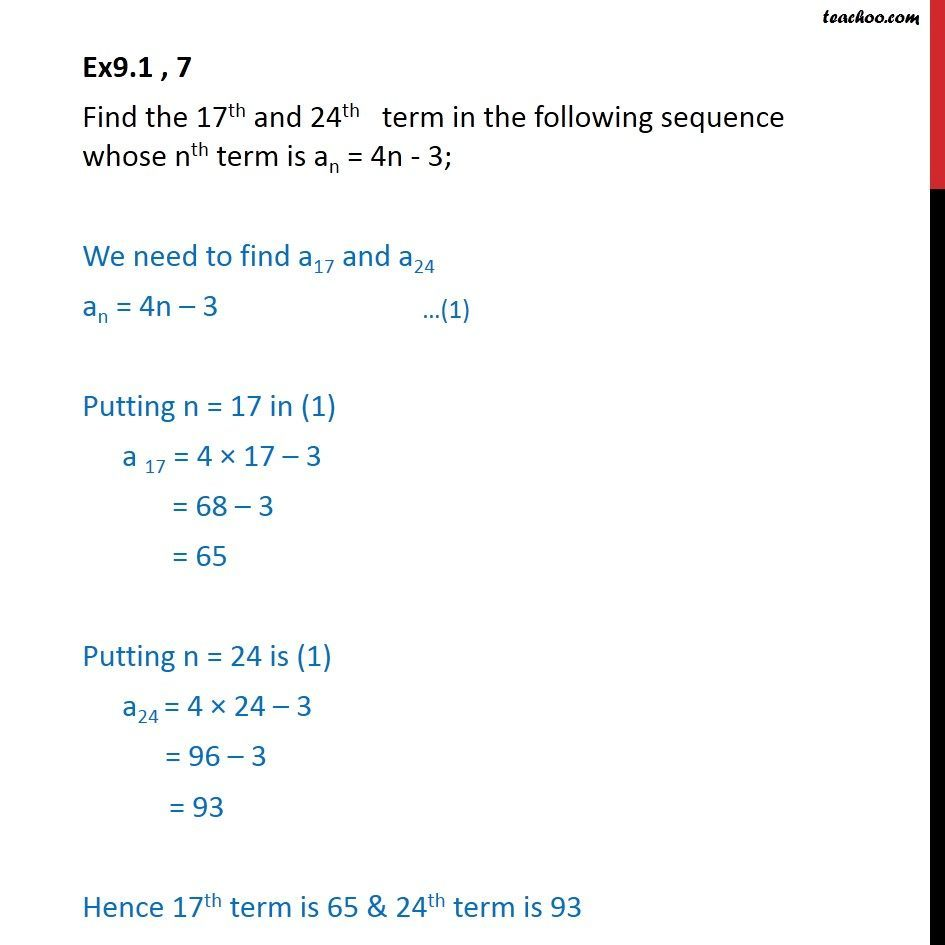Finding Sequences

Chapter 9 Class 11 Sequences and Series
Concept wiseIntroducing your new favourite teacher - Teachoo Black, at only ₹83 per month

### Transcript

Ex9.1 , 7 Find the 17th and 24th term in the following sequence whose nth term is an = 4n - 3; We need to find a17 and a24 an = 4n 3 Putting n = 17 in (1) a 17 = 4 17 3 = 68 3 = 65 Putting n = 24 is (1) a24 = 4 24 3 = 96 3 = 93 Hence 17th term is 65 & 24th term is 93